## Suppose that in the production of 60-ohm radio resistors, nondefective items are those that have a resistance between 58 and 62 ohms and the

Question

Suppose that in the production of 60-ohm radio resistors, nondefective items are those that have a resistance between 58 and 62 ohms and the probability of a resistor’s being defective is The resistors are sold in lots of 200, with the guarantee that all resistors are nondefective. What is the probability that a given lot will violate this guarantee? (Use the Poisson distribution.)

in progress 0
6 months 2021-07-24T18:53:12+00:00 1 Answers 42 views 0

## Answers ( )

The probability that a given lot will violate this guarantee is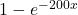, in which x is the probability of a resistor being defective.

Step-by-step explanation:

In a Poisson distribution, the probability that X represents the number of successes of a random variable is given by the following formula: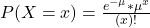In which

x is the number of sucesses

e = 2.71828 is the Euler number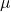is the mean in the given interval.

The probability of a resistor’s being defective is x:

This means that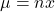, in which n is the number of resistors.

The resistors are sold in lots of 200

This means that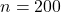, so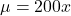What is the probability that a given lot will violate this guarantee?

This is: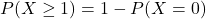In which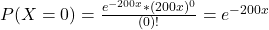So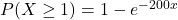The probability that a given lot will violate this guarantee is, in which x is the probability of a resistor being defective.